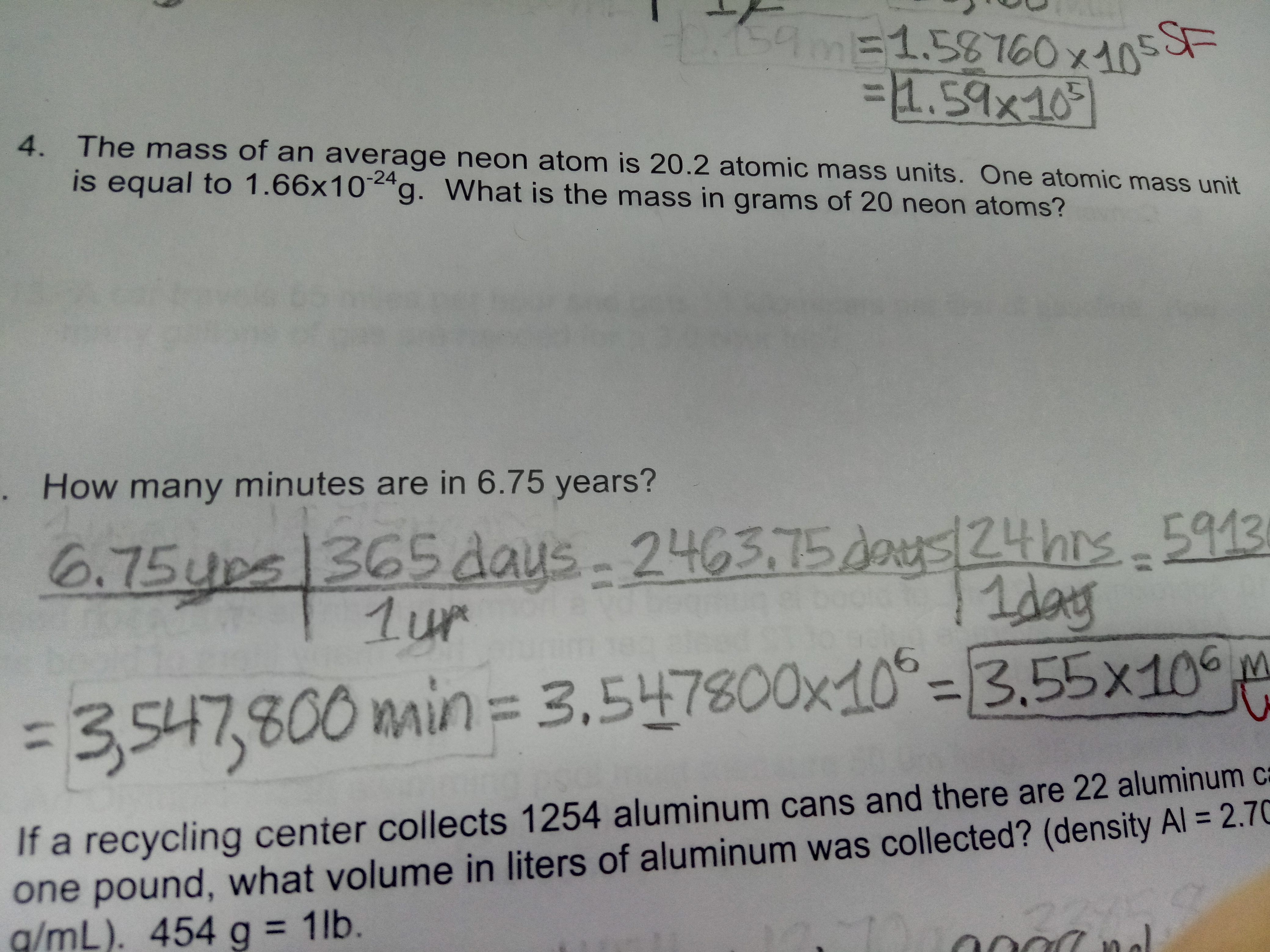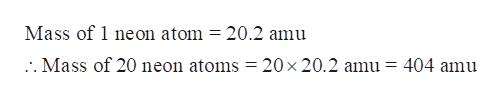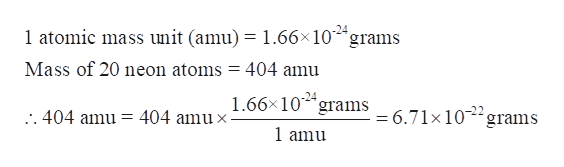# 159m-1.58T60x10F1.59x1094. The mass of an ave rage neon atom is 20.2 atomic mass units. One atomic mass unitis equal to 1.66x10 g. What is the mass in grams of 20 neon atoms?. How many minutes are in 6.75 years?24hrs 9136.75ups 365 days1ur2463.75daMAU=3.547,800 main = 3,547800x10-3.55x10If a recycling center collects 1254 aluminum cans and there are 22 aluminum caone pound, what volume in liters of aluminum was collected? (density Al = 2.70g/mL). 454 g = 1lb.

Question

I need help with #4.help_outlineImage Transcriptionclose159m- 1.58T60x10 F 1.59x109 4. The mass of an ave rage neon atom is 20.2 atomic mass units. One atomic mass unit is equal to 1.66x10 g. What is the mass in grams of 20 neon atoms? . How many minutes are in 6.75 years? 24hrs 913 6.75ups 365 days 1ur 2463.75da MAU =3.547,800 main = 3,547800x10-3.55x10 If a recycling center collects 1254 aluminum cans and there are 22 aluminum ca one pound, what volume in liters of aluminum was collected? (density Al = 2.70 g/mL). 454 g = 1lb. fullscreen
check_circleExpert Solution
Step 1

We have to calculate the mass in grams of 20 neon atoms given that

Mass of one average neon atom = 20.2 atomic mass unit (amu)

1 atomic mass unit = 1.66×10-24 grams

Step 2

Calculate the mass of 20 neon atoms in atomic mass unit.help_outlineImage TranscriptioncloseMass of 1 neon atom = 20.2 amu .. Mass of 20 neon atoms = 20 x 20.2 amu = 404 amu fullscreen
Step 3

Convert the mass of 20 neon at...help_outlineImage Transcriptionclose1 atomic mass unit (amu) = 1.66x10 grams Mass of 20 neon atoms = 404 amu 1.66x10 grams 6.71x10 grams -24 404 amu x . 404 amu = 1 amu fullscreen

### Want to see the full answer?

See Solution

#### Want to see this answer and more?

Solutions are written by subject experts who are available 24/7. Questions are typically answered within 1 hour*

See Solution
*Response times may vary by subject and question
Tagged in

### Chemistry